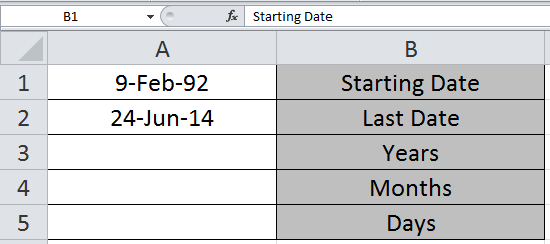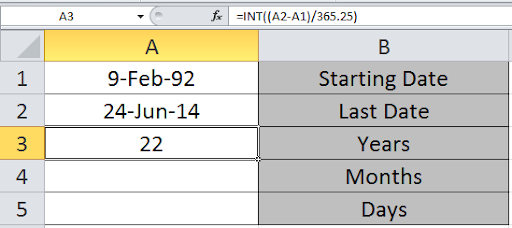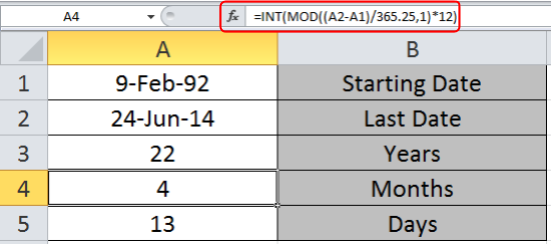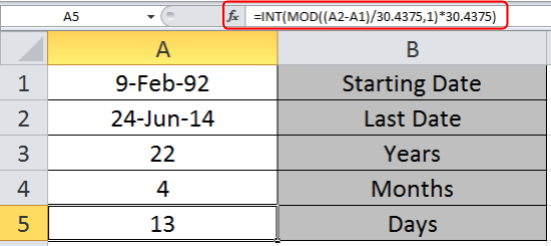# Calculating Number of Days, Months and Years between Dates in Microsoft Excel

While working on Excel, Sometimes you need to calculate the number of Days, Months and Years between the two given dates.

To calculate the number of Days, Months and Years between the two given dates, we will use INT and MOD function in Excel 2016.

INT function returns the Integer part of the number.

Syntax:

 =INT(number)

MOD function returns the remainder of the number after dividing it with the divisor

Syntax:

 =MOD(number, divisor)

Let’s get this by an example shown below.We need to find the years, month and days between the two given dates in A1 and A2 cell.

Write the formula in A3 cell to get the years between these two dates.

Formula

 =INT((A2-A1)/365.25)We can see the number of years in the A3 cell.

Now write the formula in A4  to get the months

 =INT(MOD((A2-A1)/365.25,1)*12)

Use the Formula in A5 cell to get the days between the two dates.

 =INT(MOD((A2-A1)/30.4375,1)*30.4375)

Explanation:
As we know there are 12 months in a year and an average of 365.25 days in 4-year interval.
Here INT function returns the Integer part of the mathematical formulation part.Here as you can see you get the number of Days, Months and Years between the two given dates.

Previously in Excel 2010 and Excel 2013, MS used to provide a date function named DATEDIF. It was found that it had some errors and was prone to give wrong outputs. This is why in Excel 2016 it is not promoted. But the function is still useful to calculate age. When you type this formula, no help or suggestion will be shown. You have to type it whole...

DATEDIF Syntax

Syntax:

 =DATEDIF(date1,date2, “Y”)

“Y” will return the difference of years. At the place “Y” we can also write “M” or “D”.
As you must have guessed that “M” will return the difference in Months and “D” will return difference of Days.

These basic formulas and its uses can improve your time and efficiency using MS Excel 2016. Hope you understood how to count the number of days between the two given dates. You can perform these tasks in Excel 2013 and 2010. Please share your views on this topic below in the comment box. We will help you.

Related Articles:

Calculate years between dates

How to Add Years to a Date

Convert Date and Time from GMT to CST

Get days between dates in Excel

Popular Articles:

50 Excel Shortcut to Increase Your Productivity

The VLOOKUP Function in Excel

COUNTIF in Excel 2016

How to Use SUMIF Function in Excel

## Users are saying about us...

1.Hi there,
i copy-paste your formula as you written above:
=INT((TODAY()-A1)/365.25) & ” years , ” & INT(MOD((TODAY()-A1)/365.25,1)*12) & ” months and ” & INT(MOD((TODAY()-A1)/30.4375,1)*30.4375) & ” days”

but it didnt work out. could you give me the correct formula for the result to count years, month, weeks, and days?

•Milla,

I think you adding "&" function with space may that could be reason for error, could you pls use below and let us know

=INT((TODAY()-A1)/365.25)&" years , "&INT(MOD((TODAY()-A1)/365.25,1)*12) &" months and "&INT(MOD((TODAY()-A1)/30.4375,1)*30.4375)&" days only"

thanks Rishi

•=INT((TODAY()-A1)/365.25) & " years , " &
INT(MOD((TODAY()-A1)/365.25,1)*12) & "
months and " & INT(MOD((TODAY()-A1)/30.4375,1)*30.4375) & " days"

•I think formula is not right, I try to calculate below dates
start date 12-02-1991
end date 31-01-2020
output is -29 years , 0 months and 12 days
But it should be 28 years , 11 months and 17 days

•Hi Simant,

Please check the end date & start date reference as it looks like the arguments may be disordered. You can also use the ABS function along with formula to returns a positive result.

2.INT((TODAY()-A1/365.25) & "years, " & INT(MOD((TODAY()-A1/365.25,1)*12) &" moths and "& INT(MOD((TODAY()-A1/30.4375,1)*30.4375) &"days only" , i used this formula but don't calculate, plz give me a reply

•=INT((TODAY()-A1)/365.25) & " years , " & INT(MOD((TODAY()-A1)/365.25,1)*12) & " months and " & INT(MOD((TODAY()-A1)/30.4375,1)*30.4375) & " days"

Use this. I retyped the quotation marks and it worked.

3.I think part of the problem is trying to put everything into one cell. I split it into three cells and it works fine.

A3: =INT((TODAY()-A1)/365.25)
A4: =INT(MOD((TODAY()-A1)/365.25,1)*12)
A5: =INT(MOD((TODAY()-A1)/30.4375,1)*30.4375)

4.Hi
How Do you Convert years, months, days back
to complete days

A2 = 2 years
B2 = 6 Months
C2 = 27 Days

= ? how many actual Days

•Hi Nico

•i used for converting but it giving the wrong answer

•hey,

5.Dear Sir/Mam,

I have a date in a cell like:-(03/13) i want it like: "March-13" is it possible please help me on this.

•You can select the cell where the date is in (03/13) format. Right click on the cell and select format cells. In Number Tab —> click on Date —> Select March – 13. Click on Ok

6.i'M TRYING TO SEE IF YOU CAN MAKE A FOMULA WITH A START DATE TO CALCULATE YEAR MONTH DAYS AND WILL STOP WHEN YOU ADD A STOP DATE. COULD YOU PLESE HELP ME WITH THIS PROBLEM. THANK YOU

•IN ONE CELL START DATE-THE YEAR MONTH DAYS-STOP DATE

7.I am trying to calculate number of open days on action items. Cells used for calculation are today's date, assigned date, target due date, date closed.

If there is no date closed and the action item is still open, I would like to know based on today's date how many days has the action item been open since the assigned date but if the action item has a date closed then I would like to calculate days open based on assigned date and date closed.

8.hello,
I have one date in a cell and in a blank cell i would like to know the number of days between that date and todays dates (that the workbook is open) is that possible?

•Hi Isabella

This is possible, you can do it.

9.Thank you, it works.

10.I am trying to calculate the number of months for a project end date occurring in 2014. The project start AND end date can be <2014 or greater than = 2015 - If so, the result is "0". How can I calculate the number of months in 2014 for projects that started before or during 2014?
So far I have the following:
=IF(OR(YEAR(End Date field )=2015),"",IF(YEAR(End Date field)>=2015,12,MONTH(End Date field)))

11.There seems to be one thing missing from all the formulas above which is how to calculate the weeks. I need to state the months, weeks and days between two dates on my invoice. Can anybody shed some light on getting that specific formula?

•Hi Shai,

To count the number of weeks in between two dates divide the number of days to 7.

12.Can any one tell me how i can calculate Days, Months & Year from a figure like 45. Assuming month is always of 30 days. I want result like this: 0 Years, 1 Month 15 Days.

•Hi Sajid,

•Check if this works

=ROUNDDOWN(U157/30,0)&" Month and " & MOD(U157,30)&" days"

13.Dear All,

I hope if someone here can help me with a formula where I want to get how many days it is due. I want to subtract due dates with the current day and keeps on updating or incrementing with 1 on the next day. And, using Excel 2013

Thanks to all who can help me with this in advance 🙂

Regards,
Flava

14.How can I calculate the multiple months & years: -
S. No Company From To Duration
1 Current Com. Sep-11 Mar-15 3 years, 6 months
2 Previous 1 Apr-10 May-11 1 years, 1 months
3 Previous 2 Jul-09 Dec-09 0 years, 5 months
4 Previous 3 Jun-08 Jul-09 1 years, 1 months

Total Experience ?

•Hi Raj,

Thanks

15.colin.larkworthy

Hi im trying to figure out a date of birth and subtract date x to = × months as a formulae any ideas

16.How can i calculate the week via current or previous date. If any suggestion please reply.

17.kunjumol shaji

How I calculate no.of years experience from joining date to current date.

18.How would I calculate the exact months and exact days between two dates in excel?

Thanks,

Terms and Conditions of use

The applications/code on this site are distributed as is and without warranties or liability. In no event shall the owner of the copyrights, or the authors of the applications/code be liable for any loss of profit, any problems or any damage resulting from the use or evaluation of the applications/code.